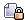###Author Topic: Calculator  (Read 31882 times)

####*Armageddon

• Single posting newcomer
•• Posts: 8##### Calculator
« on: July 19, 2010, 09:23:58 am »
Hello allI have been slowly learning C++, and one thing that i had been itching to do was to make a calculator.

So far i have:
#include <iostream>

using namespace std;

int main()
{
int digit1;
cout << "Enter your first digit, and then press enter" << endl;
cin >> digit1;
int digit2;
cout << "Enter your second digit, and then press enter" << endl;
cin >> digit2;
int o;
cout << "If you wish to add, press 1, and then enter" << endl;
cout << "If you wish to suntract, press 2, and then enter" << endl;
cout << "If you wish to multiply, press 3, and then enter" << endl;
cout << "If you wish to divide, press 4, and then enter" << endl;
cin >> o;
if (o = 1)
{
cout << "The sum of your two digits is " << digit1+digit2 << endl;
}

else if (o = 2)
{
cout << "The difference of your two digits is " << digit1-digit2 << endl;
}

else if (o = 3)
{
cout << "The product of your two digits is " << digit1*digit2 << endl;
}

else if (o = 4)
{
cout << "The quotient of your two digits is " << endl;
cout << "Quotient " << digit1/digit2 << endl;
cout << "Remainder " << digit1%digit2 << endl;
}

else if (o > 4)
{
cout << "Invalid operation" << endl;
}
return 0;
}

My main problem is that i can only get the sum of the two digits...any help here?
My other question was if i could some how change the 1, 2, 3, 4 to +,-,*,/

Help would be greatly appreciated####*Armageddon

• Single posting newcomer
•• Posts: 8##### Re: Calculator
« Reply #1 on: July 19, 2010, 09:30:37 am »
Actually, i solved my first problem by changing (example = 1) to (example > 0 && example < 2)
Is this a good way to do it? or should i have done something else?

####killerbot

• Lives here!
•• Posts: 5295##### Re: Calculator
« Reply #2 on: July 19, 2010, 10:29:04 am »
hello, nice to see someone starting with C++.
However these forums are related to use of CB, it is not a general programming forum.
So you will have to look elsewhere, but let's give you some feedback though :

all your tests like : if (0 = 5), these are not the tests you intended, you just do assignments : o = 5, whose result is '5' so you actually wrote if(5).
It has to be 2 = signs --> ==    : if (0 == 5)

Other suggestions : transform those 1, 2, 3 into an enumeration with a good name
enum MyOperations
{